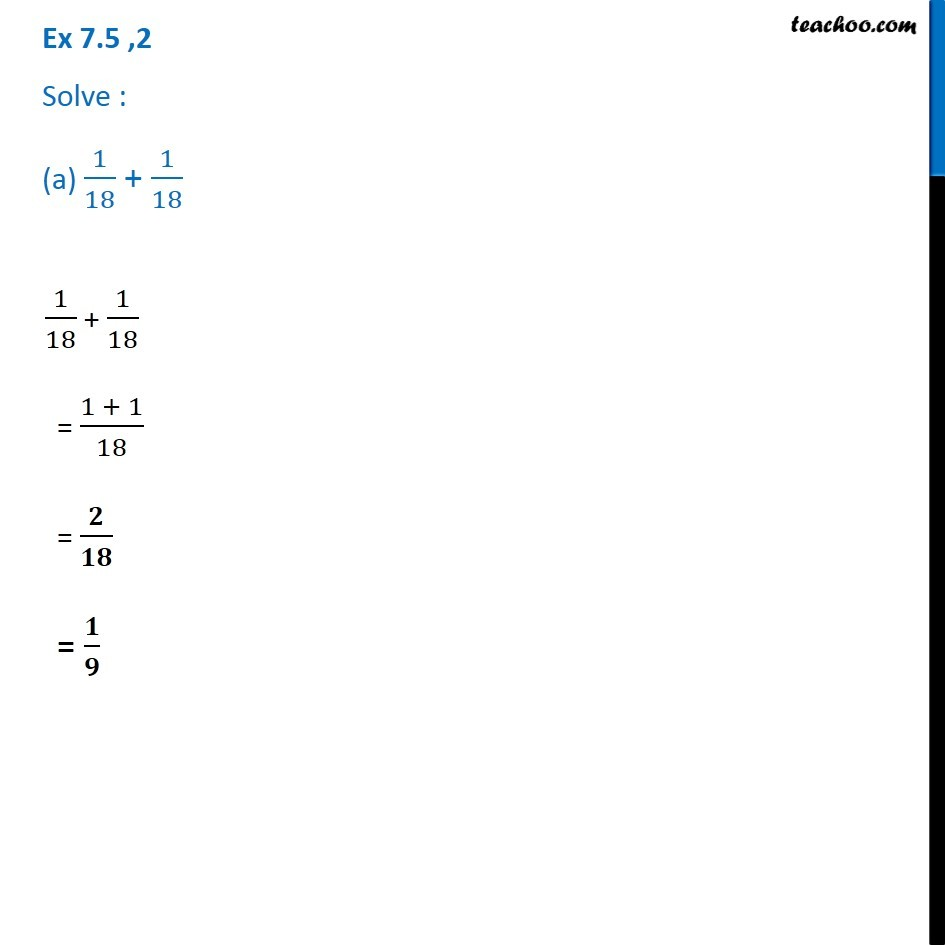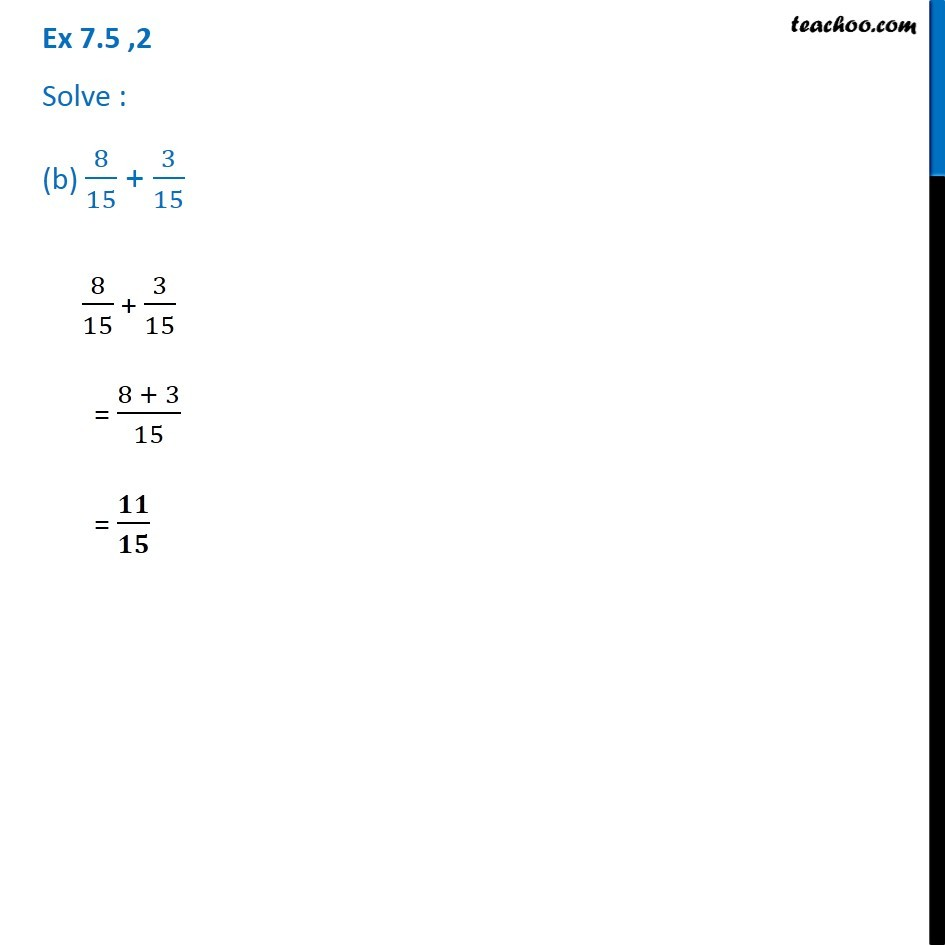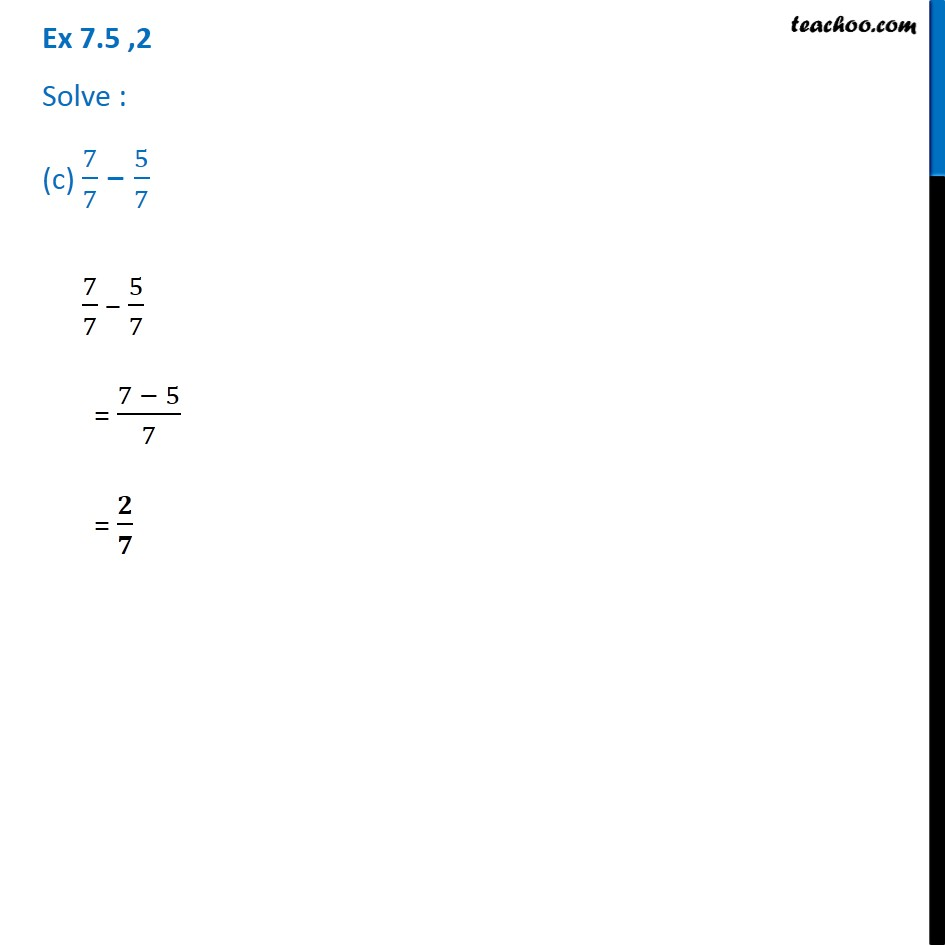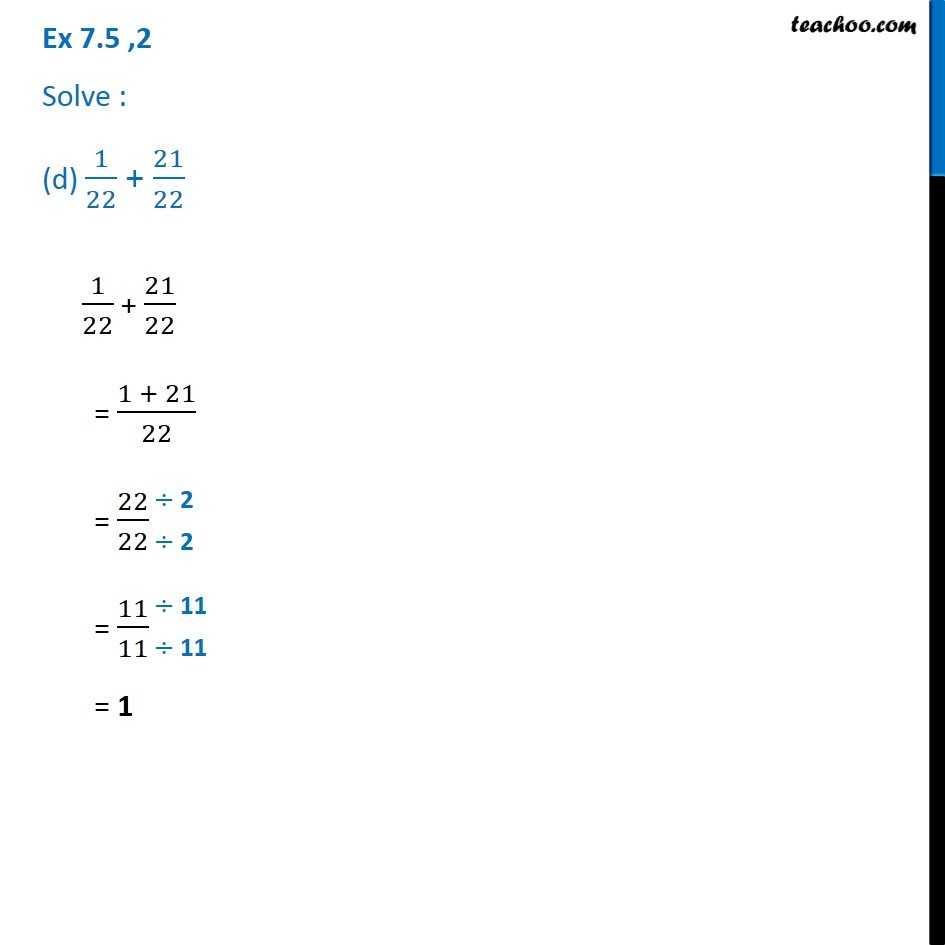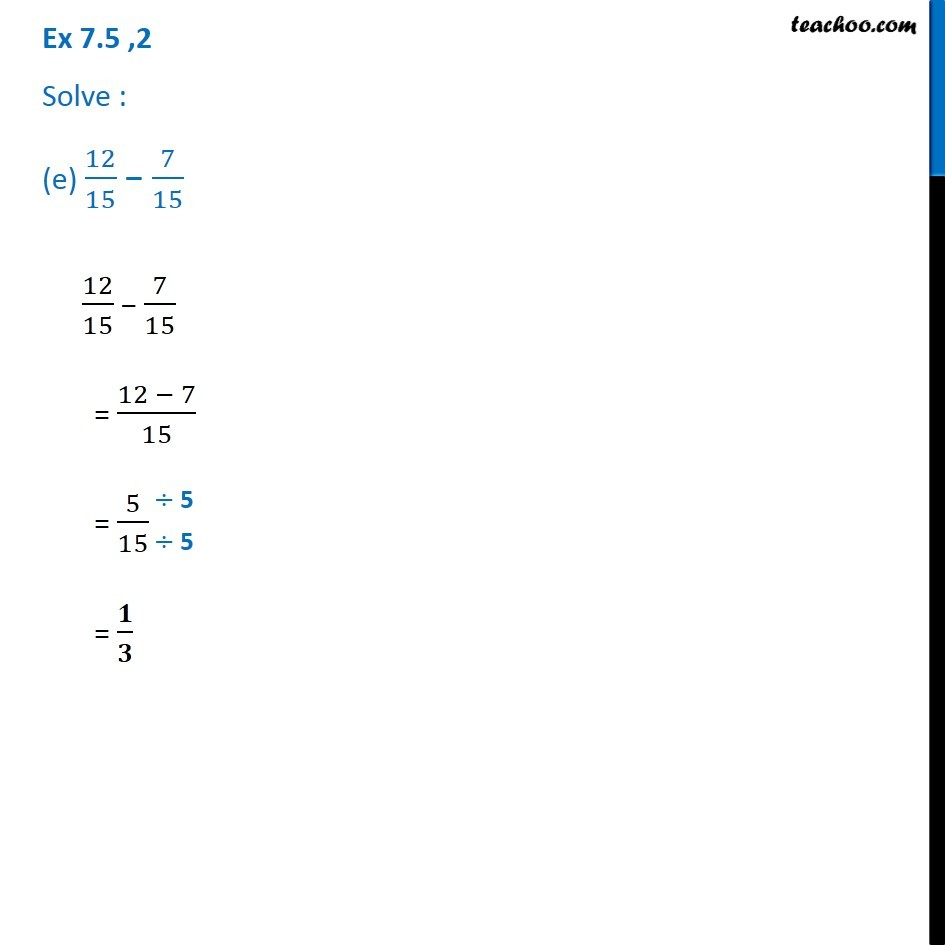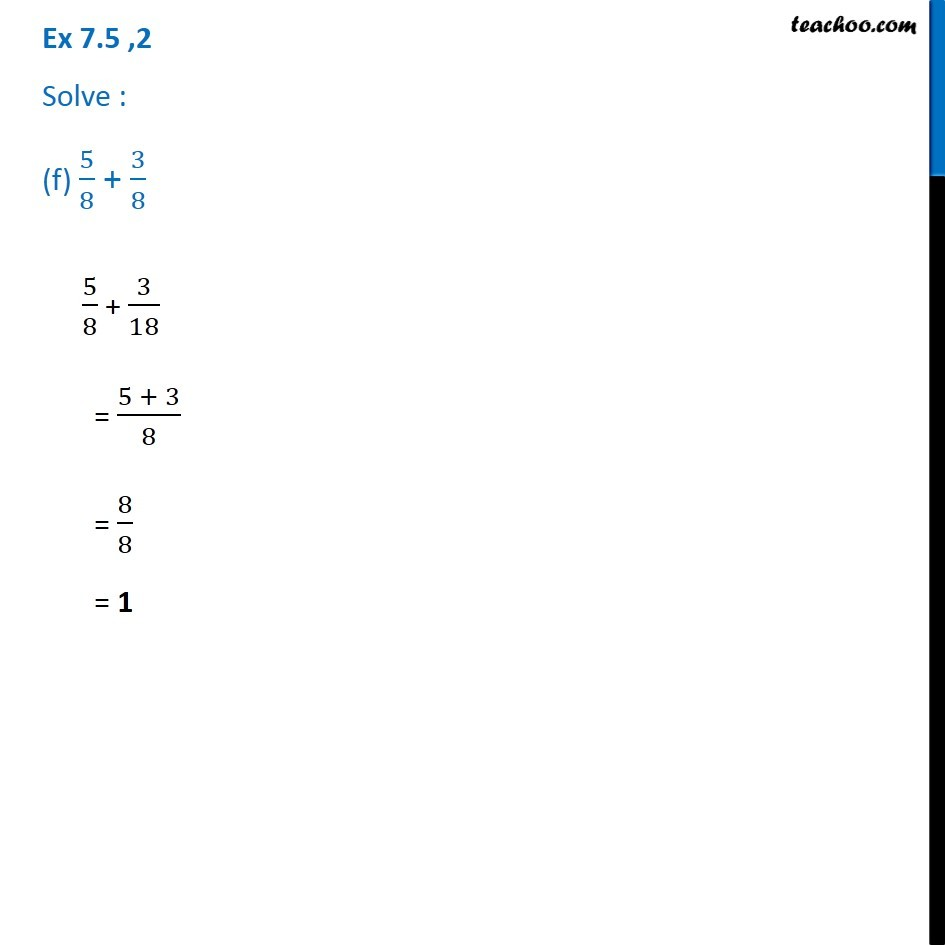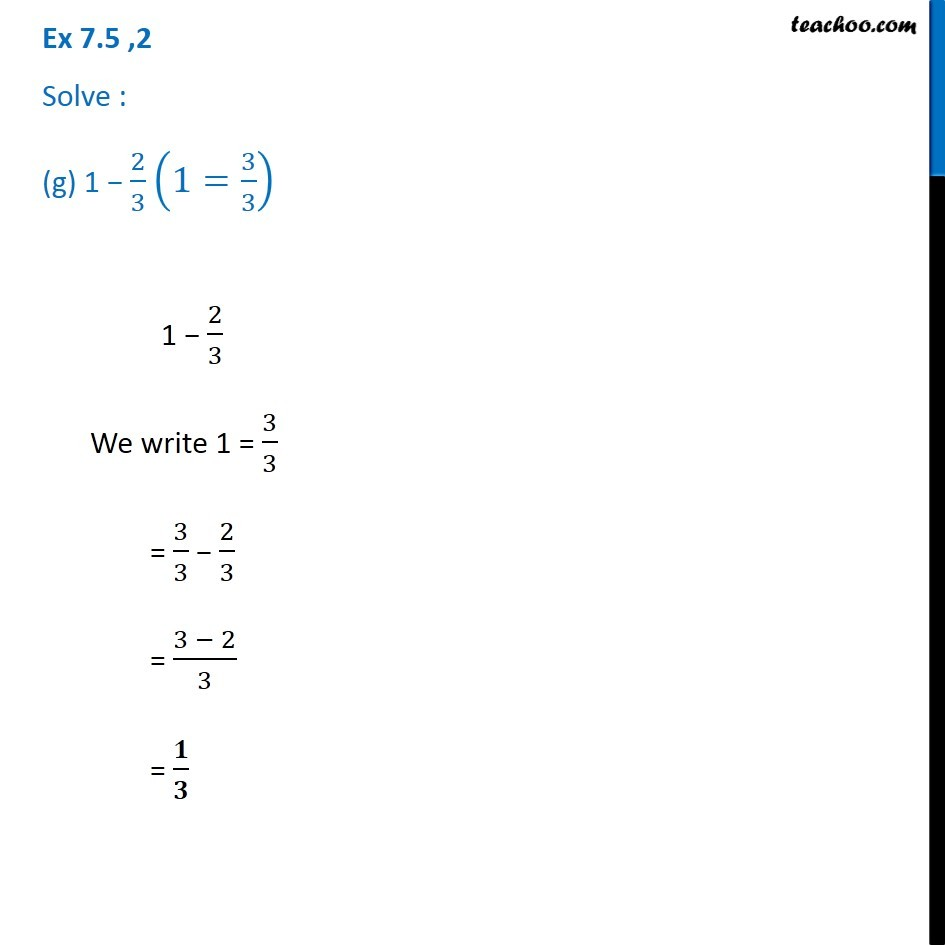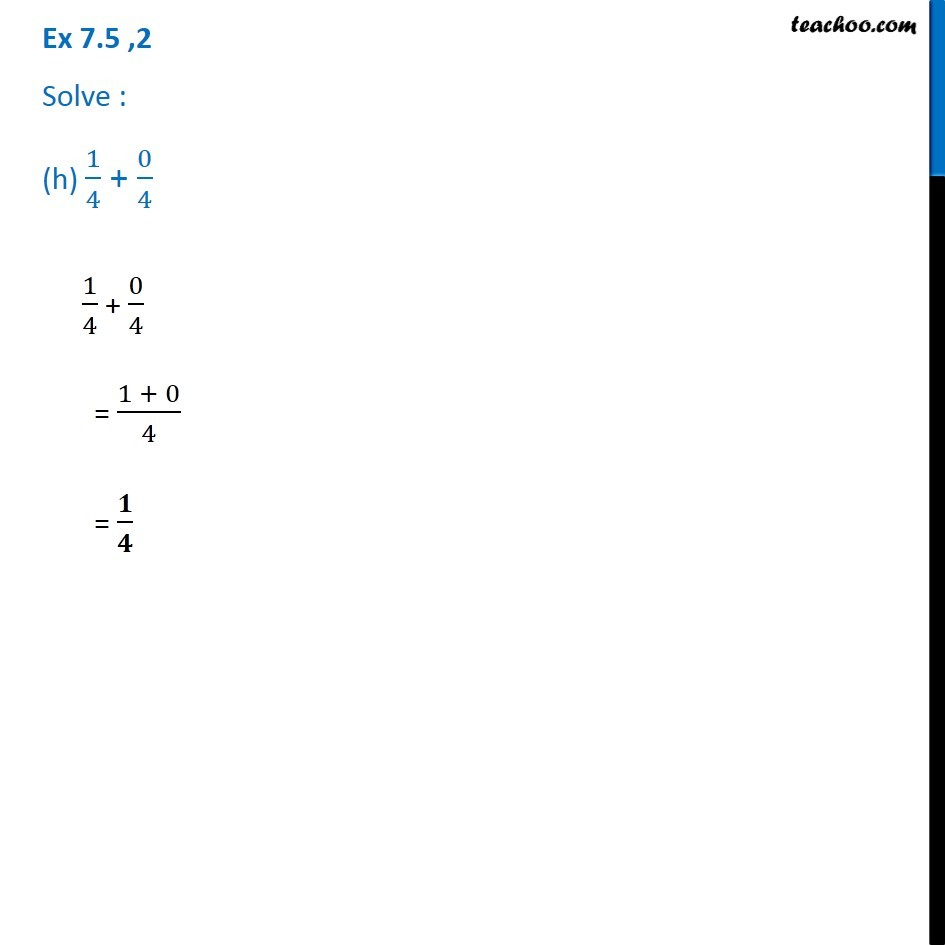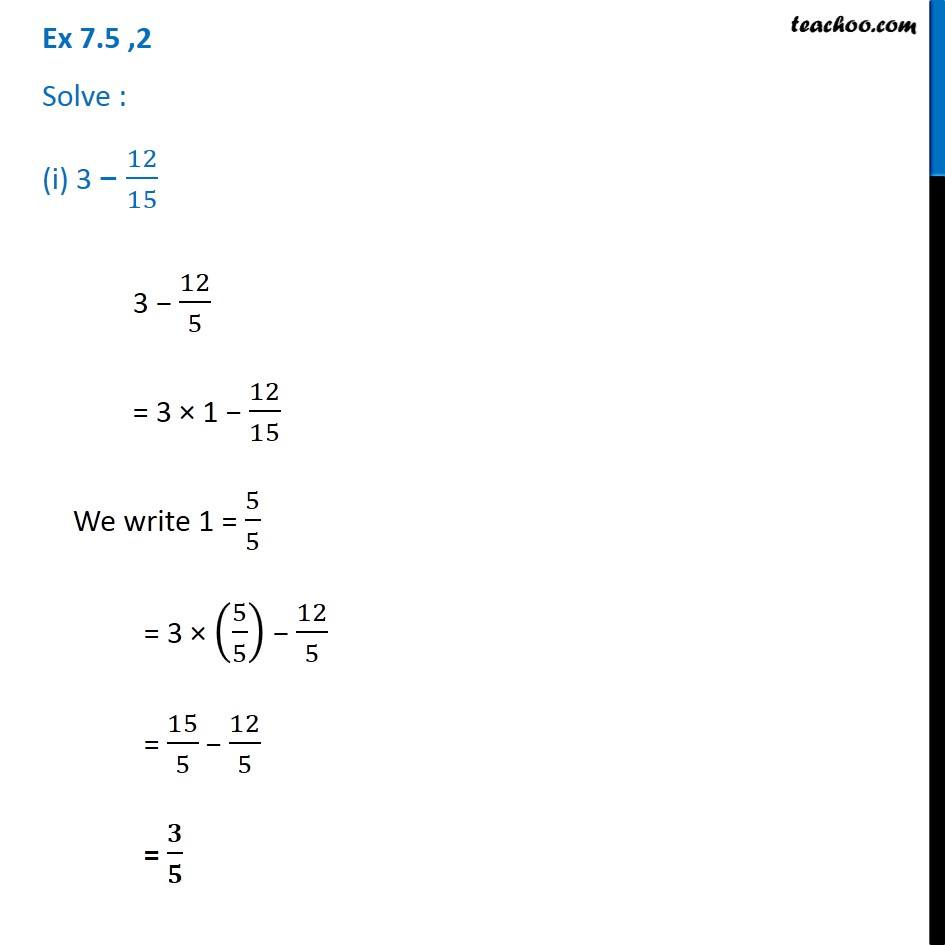Subscribe to our Youtube Channel - https://you.tube/teachoo

1. Chapter 7 Class 6 Fractions
2. Serial order wise
3. Ex 7.5

Transcript

Ex 7.5 ,2 Solve : (a) 1/18 + 1/181/18 + 1/18 = (1 + 1)/18 = 𝟐/𝟏𝟖 = 𝟏/𝟗 Ex 7.5 ,2 Solve : (b) 8/15 + 3/158/15 + 3/15 = (8 + 3)/15 = 𝟏𝟏/𝟏𝟓 Ex 7.5 ,2 Solve : (c) 7/7 − 5/77/7 − 5/7 = (7 − 5)/7 = 𝟐/𝟕 Ex 7.5 ,2 Solve : (d) 1/22 + 21/221/22 + 21/22 = (1 + 21)/22 = 22/22 = 11/11 = 1 Ex 7.5 ,2 Solve : (e) 12/15 − 7/1512/15 − 7/15 = (12 − 7)/15 = 5/15 = 𝟏/𝟑 Ex 7.5 ,2 Solve : (f) 5/8 + 3/85/8 + 3/18 = (5 + 3)/8 = 8/8 = 1 Ex 7.5 ,2 Solve : (g) 1 − 2/3 (1=3/3) 1 − 2/3 We write 1 = 3/3 = 3/3 − 2/3 = (3 − 2)/3 = 𝟏/𝟑 Ex 7.5 ,2 Solve : (h) 1/4 + 0/41/4 + 0/4 = (1 + 0)/4 = 𝟏/𝟒 Ex 7.5 ,2 Solve : (i) 3 − 12/153 − 12/5 = 3 × 1 − 12/15 We write 1 = 5/5 = 3 × (5/5) − 12/5 = 15/5 − 12/5 = 𝟑/𝟓 3 − 12/5 = 3 × 1 − 12/15 We write 1 = 5/5 = 3 × (5/5) − 12/5 = 15/5 − 12/5 = 𝟑/𝟓

Ex 7.5

Chapter 7 Class 6 Fractions
Serial order wise